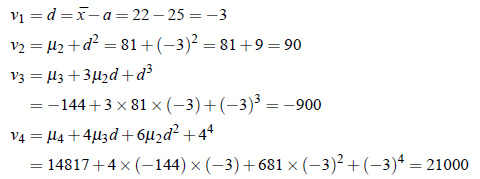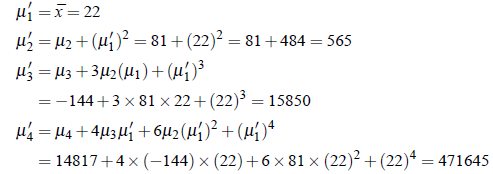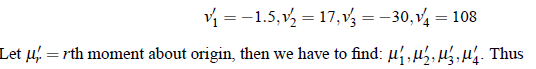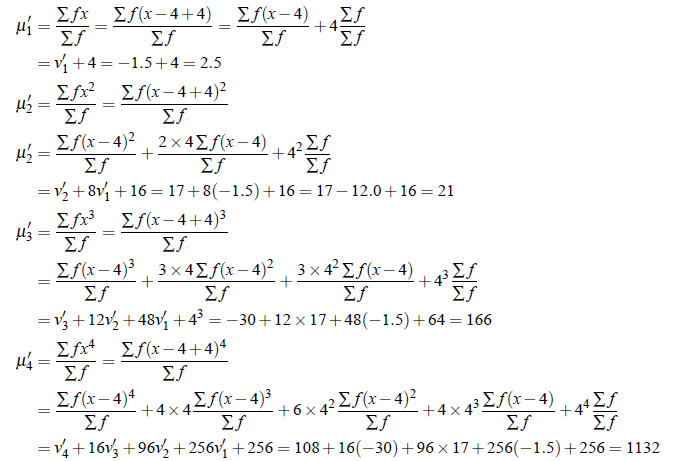Method I: Convert the moments about a in terms of moments about mean and then obtain moments about origin.

Method II: Obtain by the formula.

Example:1 The arithmetic mean of a series is 22 and first four central moments are 0,81,-144 and 14817. Find the first four moments (i) about assumed 25 and (ii) about origin.

Solution:
(i) Moments about assumed mean a = 25: